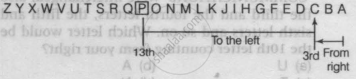# If the English Alphabet Series is Written in Backward Order, Then What Will Be the 13th Letter to the Left of the 3rd Letter from Right? - Logical Reasoning

MCQ

If the English Alphabet series is written in backward order, then what will be the 13th letter to the left of the 3rd letter from right?

• P

• N

• R

• Q

#### Solution

P
Explanation:
Backward order is written asTherefore, the 13th letter to the left of the 3rd letter from right is P.

Alternate method
In backward order of alphabet, 13th letter to the left of 3rd letter from right = (3 + 13)th letter from right = 16th from right = P

Concept: Alphabet and Numeric Test
Is there an error in this question or solution?